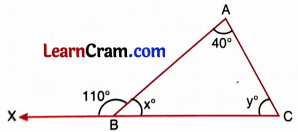# DAV Class 7 Maths Chapter 8 Worksheet 2 Solutions

The DAV Maths Book Class 7 Solutions Pdf and DAV Class 7 Maths Chapter 8 Worksheet 2 Solutions of Triangle and Its Properties offer comprehensive answers to textbook questions.

## DAV Class 7 Maths Ch 8 WS 2 Solutions

Question 1.
In ΔABC, ∠A = 44°. If AB = AC, find ∠B and ∠C.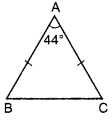AB = AC [given]
∠B = ∠C
∠A + ∠B + ∠C = 180° [Angles sum property]
44° + ∠B + ∠B = 180°
2∠B = 180° – 44°
2∠B = 136°
∠B = $$\frac{136^{\circ}}{2}$$ = 68
∠B = ∠C = 68°.

Question 2.
ΔPQR is an isosceles triangle with PQ = PR. If ∠R = 42°, find the measure of ∠P.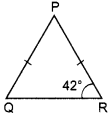PQ = PR [given]
∠Q = ∠R [Angles opposite to equal sides]
But ∠R = 42°
Q = 42° [given]
∠P + ∠Q + ∠R = 180° [Angles sum property]
∠P + 42° + 42° = 180°
∠P + 84° = 180°
∠P = 180° – 84°
∠P = 96°
∠P = 96°.Question 3.
ΔABC is an isosceles triangle with AB = AC. If ∠B of ∠A and ∠C?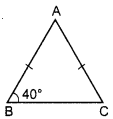AB = AC [given]
∠B = ∠C [Angles opposite to equal sides]
But ∠B = 40° [given]
∠C = 40°
∠A + ∠B + ∠C = 180°
∠A + 40° + 40° = 180°
∠A + 80° = 180°
∠A = 180° – 80° = 100°
Hence ∠A = 100° and ∠C = 40°.

Question 4.
The vertical angle of an isosceles triangle is 100°. Find its base angles.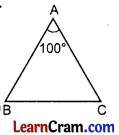∠A + ∠B + ∠C = 180° [Angle Sum Property]
⇒ 100° + ∠B + ∠C = 180°
⇒ ∠B + ∠C = 180° – 100°
⇒ ∠B + ∠C = 80°
But ∠B = ∠C (Base angles of an isosceles triangle)
∠B = ∠C = 40°

Question 5.
In given figure, ΔABC is isosceles with AB = AC. Find the values of x and y.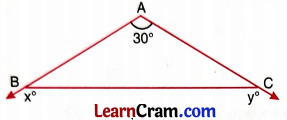In ΔABC,
AB = AC
∠B = ∠C
∠A + ∠B + ∠C = 180°
30° + ∠B + ∠B = 180°
2∠B = 180° – 30° = 150°
∠C = 75°
∠B + ∠x = 180° [Linear pairs]
75° + x = 180°
x = 180° – 75° = 105°
∠C + ∠y = 180° [Linear Pair]
75° + ∠y = 180°
∠y = 180° – 75° = 105°
Hence ∠x = ∠y = 105°.Question 6.
In given figure, find ∠ACB and ∠A and write which sides of ΔABC are equal?∠ACD + ∠ACB = 180°
130° + ∠ACB = 180°
∠ACB = 180° – 130° = 50°
∠A + ∠B + ∠ACB = 180° [Angle Sum Property]
∠A + 80° + 50° = 180°
∠A = 180° – 80° – 50°
= 180° -130°
= 50°
∠A = 50°.

Question 7.
In given figure, AB = AC, and AP ⊥ BC and ∠B = 60°. Then find (i) ∠BAP (ii) ∠ACB.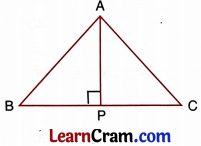AB = AC [given]
∠B = ∠C (Angle opposite to equal sides of a triangle)
∠B = 60°
∠C = 60°
∠A + ∠B + ∠C = 180° (Angle Sum Property)
∠A + 60° + 60° = 180°
∠A + 120° = 180°
∠A = 180° – 120° = 60°
(i) ∠BAP = $$\frac{1}{2}$$ ∠A = $$\frac{1}{2}$$ × 60° = 30°
(ii) ∠ACB = 60° = ∠ABC

Question 8.
In given figure, find x and y. Is ΔABC isosceles? If so, name its equal sides.